Acids and bases are important elements in organic chemistry. The presence of acids and bases allows the synthetic reactions of compounds to proceed through the movement of electrons. In other words, without acids and bases, organic synthesis reactions would not occur.

Therefore, we must understand the definitions of acids and bases in advance. We need to understand what kinds of compounds are acids and what kinds of compounds are bases.

However, whether it is an acid or a base depends on the conditions. Even if a compound is an acid, it can often become a base under other conditions. A substance that is acidic in solution is not necessarily an acid. Even substances that are generally considered to be acids can often be bases.

So let’s understand the definitions of acids and bases. We’ll first understand the Brønsted-Lowry definition, and then we’ll check how to think about Lewis acids and Lewis bases.

## High School Chemistry Is Defined by Arrhenius and Brønsted-Lowry

There are some definitions of acids and bases. One of the simplest and easiest to understand is the Arrhenius definition. Arrhenius definition of acids and bases is as follows.

• Acid: a compound that produces H+ in an aqueous solution.
• Base: a compound that produces OH in an aqueous solution.

However, this definition is useless in chemistry. In the first place, chemical reactions do not proceed only in aqueous solutions.

So the Brønsted-Lowry theory was devised. In the Brønsted-Lowry definition, acids and bases are defined as follows

• Brønsted acid: a molecule that gives H+.
• Brønsted base: a molecule that receives H+.

In high school chemistry, all we learned was the Brønsted-Lowry definition. Even when we learn about Brønsted acids and Brønsted bases, we don’t learn about the details of conjugate acids, conjugate bases, and acid strength indicators. Therefore, it is necessary to have a deep understanding of why acids and bases are generated.

Also, to understand Lewis acids and Lewis bases, it is common to learn about Brønsted acids and Brønsted bases first, so let’s see the details.

### In Acids and Bases, There Are Always Conjugate Acid and Conjugate Base

There are many molecules known as acids and bases. For example, ammonia is a base. This is because it receives H+. Water, on the other hand, is an acid because it gives H+.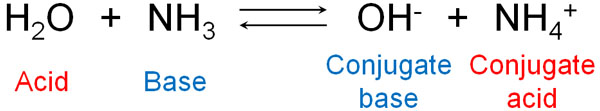The molecules that form after the acid gives H+ are called conjugate base. Since the acid gives H+, a compound with a negative charge is generated. This compound receives H+ in the reverse reaction, hence the name conjugate base.

On the other hand, the molecule that is formed after the base receives H+ is called a conjugate acid. The name conjugate acid comes from the fact that it gains a positive charge and gives H+ in the reverse reaction.

When ammonia dissolves in water to form ions, NH4+ is the conjugate acid. Consider that after the reaction, the molecule with a positive charge is a conjugate acid. On the other hand, when ammonia dissolves in water, OH, which has a negative charge, is called the conjugate base.

### Brønsted Acids and Brønsted Bases Are Relatively Involved

Are acids and bases absolute? No, acids and bases are relative. It is common for a substance to be acid at one time but a base under other conditions.

For example, water is both an acid and a base. If water reacts with hydrochloric acid (HCl), it acts as a Brønsted base. On the other hand, if it reacts with ammonia, water gives H+ and becomes an acid.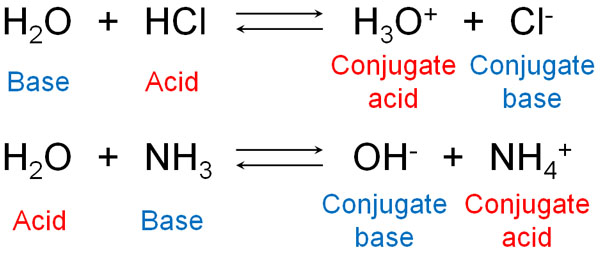Of course, many molecules, not just water, can become acids or bases.

If there is a stronger acid, the compound will act as a base. On the other hand, if you mix a strong basic reagent, the compound will act as an acid. Depending on what kind of acidic or basic reagent you use, the acid and base of the compound will be different.

For example, is an amine an acidic substance or a basic substance? Generally speaking, compounds with amino groups are considered to be basic substances. However, this is because we use water as a standard. Depending on the conditions, amines can be acids.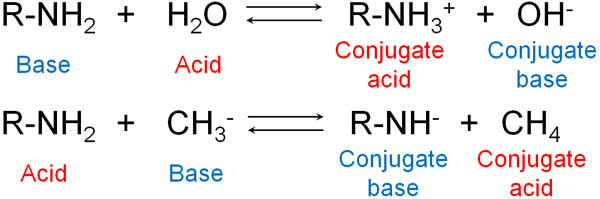When we understand this fact, we realize that there are no absolute acidic or basic substances. This is because acidity or basicity depends on what kind of reagent you use.

## A Measure of Acid Strength: pKa Value

How do we express the strength of an acid? The pKa value is a measure of acidity.

For example, let’s say you dissolve hydrochloric acid (HCl) in water. However, not all of it is ionized. Only some of it is ionized. In some cases, it exists as hydrochloric acid molecules, and in other cases, hydrochloric acid exists as ions and shows acidity.

The equilibrium constant (K) can be produced at equilibrium.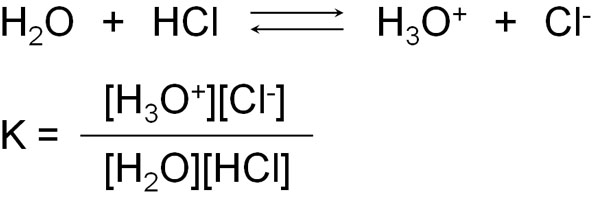However, in the equilibrium constant (K), water (H2O) is a solvent and is present in excess. And even if it reacts with hydrochloric acid, the amount of water will change only slightly because of the large amount of water present as a solvent. Therefore, we can ignore [H2O] in the above equation.

Therefore, the equilibrium constant can be written as follows, omitting [H2O].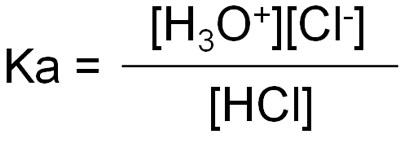However, it is difficult to understand the equilibrium constant as it is. So, we can apply pKa to the following equation using the log.

• pKa = -log Ka

The lower the pKa value, the more acidic it is. Conversely, the higher the pKa value, the more basic it is. When checking acidity and basicity, be sure to check the pKa value.

For reference, the pKa is as follows for each molecule.

 Compound pKa HI -10 HBr -9 HCl -7 HF 3.2 CH3COOH 4.8 CH3OH 15.5 H2O 15.7 HC≡CH 25 NH3 33 CH4 48

There is no need to remember the pKa values of these molecules. Just understand that there are differences in acidity between these compounds. Also, make sure that the lower the pKa value, the more acidic it is, and the higher the pKa value, the more basic it is.

### The More Stable the Conjugate Base Is, the More Acidic It Is (the Less Basicity)

When does it become acid or base? Let’s understand that this depends on the nature of the conjugate acid and the conjugate base.

Whether or not a compound is acidic depends on whether or not the conjugate base is stable. When an acid becomes an ion, it becomes negatively charged by providing H+. In other words, in an ion, a conjugate base is produced.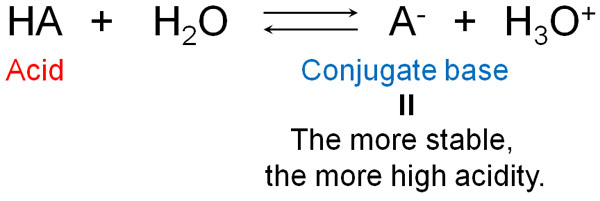When the conjugate base state is stable, the compound actively tries to provide H+. It wants to provide the H+ as soon as possible and become negatively charged.

In the same way, the strength of the base can be thought of in terms of the strength of the conjugate acid. The conjugate acid is what is produced after the base receives H+. The more stable the conjugate acid is, the more the base will try to become stable by receiving H+.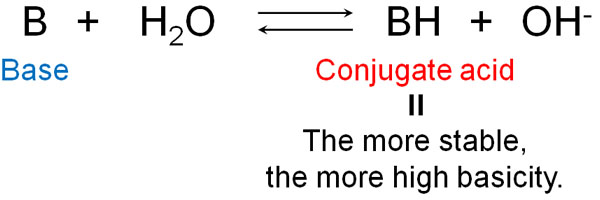Incidentally, bases always have unshared electron pairs (lone pairs). The unshared electron pair receives hydrogen, which stabilizes the conjugate acid.

As for the acidity or basicity, you need to check the pKa value. Of course, as mentioned above, there is no point in remembering the pKa value of a compound. However, by checking the stability of the conjugate acid or the conjugate base from the pKa table, you will be able to infer the acid/base reactivity of the compound.

## How Are Acidity and Basicity Determined?

How is acidity (or basicity) determined: the degree of acidity or basicity can be determined by a high or low pKa value. However, remembering the pKa value is useless; we need to understand the mechanism of why the acidity or basicity increases.

In this regard, acidity and basicity are related to the following factors.

• Electronegativity
• Size of an atom
• Resonance structures
• Hybrid orbital

There are many other factors involved in acidity and basicity. However, the main factors are these.

-The Higher the Electronegativity, the Higher the Acidity

When an atom of high electronegativity is bonded to a hydrogen atom, it causes the molecule to split into positive and negative charges. As a result, the molecule becomes polarized. Due to the high electronegativity, the higher the degree of polarization, the more the atoms attract electrons. This means that hydrogen atoms are more likely to be positively charged, resulting in a higher degree of acidity.

Compare, for example, the second-period elements. In this case, acidity is in the order of electronegativity.

• CH4 (pKa: 48) > NH3 (pKa: 33) > H2O (pKa: 15.7) > HF (pKa: 3.2)

The higher the electronegativity, the higher the acidity.

-The Larger the Atom, the Higher the Acidity

However, acidity is not the only factor involved in electronegativity. The size of the atoms is also important. For example, halogens have the following pKa.

• HF (pKa: 3.2) > HCl (pKa: -7) > HBr (pKa: -9) > HI (pKa: -10)

If we think in terms of electronegativity, the fluorine atom has the highest degree of electronegativity. However, the acidity is opposite to the order of electronegativity. This is due to the size of the atom.

The larger the atoms, the greater the bond distance from the molecules and the weaker the bond strength. As a result, it is more likely to provide H+ and become an ion. The larger the atom, the easier it is to stabilize the structure of the conjugate base.

-The Structure Is Stabilized by Resonance of the Conjugate Base

Resonance also contributes greatly to acidity (or basicity). Resonance of the conjugate base stabilizes the structure by having electrons present in various locations. This is called the delocalization of electrons. For example, the resonance structure of p-nitrophenol can be written as follows.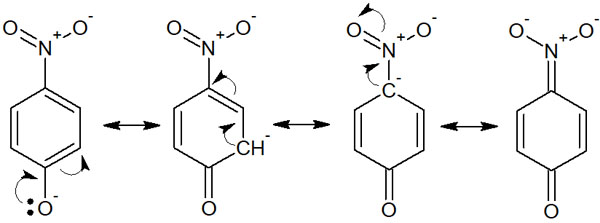It is more acidic than phenol because electrons can move to many places. This is because the conjugate base is stabilized by resonance. Similarly, when the conjugate acid is stabilized by resonance, it becomes more basic.

-The More s Character the Hybrid orbital, the More Acidic It Is

Hybrid orbitals are related to acidity. There are two types of orbitals: s-orbital and p-orbital, and the higher the percentage of s-orbital, the more acidic they are.

The s orbital is closer to the nucleus than the p orbital. Since the nucleus is positively charged, it is easier to stabilize a negative charge. In other words, when the conjugate base is negatively charged, it tends to become stable. As a result, the acidity is high.

For example, the order of acidity is as follows.

• HC ≡ CH (acetylene: pKa25)
• H2C=CH2 (ethylene: pKa44)
• CH3CH3 (ethane: pKa50)

The s-character (percentage of s-orbital) of ethane is 25%. On the other hand, the s-character of ethylene is 33%. The s-character of acetylene is 50%. Therefore, the acidity is higher in acetylene with a higher s-character.

## The Difference and Concept of Lewis Acids and Lewis Bases

So far, this is a detailed description of the definitions of acids and bases by Brønsted-Lowry. It is important in organic chemistry to understand when they become acids or bases.

However, in organic chemistry, even Brønsted-Lowry theory is not sufficient. The reason is simple: there are many synthetic reactions that do not involve H+. Therefore, we have to understand a different concept for acids and bases.

So, we will use Lewis acids and Lewis bases. Lewis acids and Lewis bases are defined as follows.

• Lewis acids: molecules that receive electrons.
• Lewis bases: molecules that provide electrons.

A Lewis acid is a Brønsted acid, and a Lewis base is also a Brønsted base. For example, the reaction is as follows.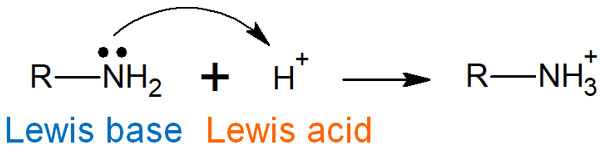In synthetic organic chemistry, chemical reactions are often described by electron arrows. Electrophilic reagents are used as reagents that receive electrons. The electrophilic reagent is a Lewis acid.

On the other hand, there are reagents that attack other compounds. These reagents are called nucleophilic reagents. Nucleophilic reagents are Lewis bases, and it is known that the stronger the basicity, the stronger the nucleophilic property. With Lewis acids and Lewis bases, we can think of reactions using acids and bases even when H+ is not involved.

### How to Distinguish Between Electrophilic and Nucleophilic Reagents

How do we distinguish between a Lewis acid (electrophilic reagent) and a Lewis base (nucleophilic reagent)?

The way to distinguish them is simple: when you draw an arrow in a chemical reaction, the molecule that accepts electrons is a Lewis acid. The more acidic a compound is, the more capable it is of accepting electrons.

When we understand this, we can consider compounds with empty orbitals to be acids, such as the following.

• Boron (B)
• Aluminum (Al)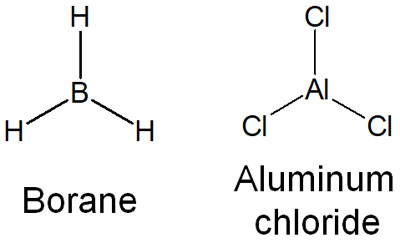Why do boron and aluminum become Lewis acids? Because they have an unoccupied orbital that allows them to accept electrons. That’s why boron (borane: BH3) and aluminum (aluminum chloride: AlCl3) are known to be strong Lewis acid catalysts.

Metals are often used in Lewis acids. Metal elements have unoccupied orbitals, which often result in Lewis acids that accept electrons.

Compounds with unoccupied orbitals do not attack other molecules and make new bonds. Therefore, they can be distinguished as Lewis acids.

-Distinguish Between Lewis Bases

On the other hand, what about Lewis bases? In nucleophilic reagents, they will always have unshared electron pairs (lone pairs). Think of any compound that has strong nucleophilicity (strong basicity) as having unshared electron pairs.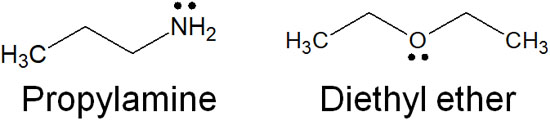Even if the lone pair is present, it can become acid. In fact, although amines are generally basic, in the presence of a stronger base, the amine can become acid.

But without an unshared electron pair, they cannot be nucleophiles. Understand that in order to give electrons, the presence of electrons that are not involved in the bond is essential. This is how you should distinguish between Lewis acids (electrophilic reagents) and Lewis bases (nucleophilic reagents).

## There Are Definitions, Types, and Characteristics of Acids and Bases

In organic chemistry, most reactions are carried out by acids and bases. All of these compounds have acidity and basicity, and by studying the conjugate acids and bases, we will be able to distinguish the difference in acidity (pKa).

Acids and bases by Brønsted-Lowry definition are relative. Depending on what reagent you mix with it, it will be acids in some conditions and bases in others. With acids and bases, you have to recognize this fact.

However, in chemical reactions, H+ doesn’t necessarily move. Therefore, it is not enough to think only in terms of Brønsted acids and Brønsted bases. So we have to learn the concept of Lewis acids and Lewis bases. You can distinguish between acids and bases depending on whether they receive or give electrons.

Learning these differences will help you to understand organic chemistry better. Understanding acids and bases are the first steps in organic chemistry, so be sure to learn the concepts.